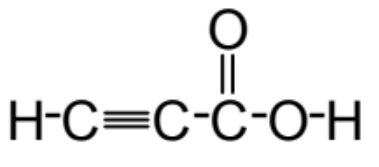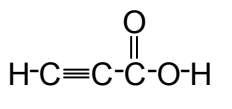# Problem: In the Lewis structure below, correctly identify the number of sigma bonds and number of pi bonds.A) six sigma, two piB) seven sigma, three piC) six sigma, three piD) four sigma, two piE) NONE of the statements above correctly identifies the number of sigma and pi bonds

###### FREE Expert Solution

We are asked to count how many sigma and pi bonds are in the lewis structure.The number of pi and sigma bonds varies depending on the type of bond.

Recall:

Single bond: 1 sigma bond
Double bond: 1 sigma + 1 pi bond
Triple Bond: 1 sigma + 2 pi bond

97% (301 ratings)###### Problem Details

In the Lewis structure below, correctly identify the number of sigma bonds and number of pi bonds.A) six sigma, two pi

B) seven sigma, three pi

C) six sigma, three pi

D) four sigma, two pi

E) NONE of the statements above correctly identifies the number of sigma and pi bonds